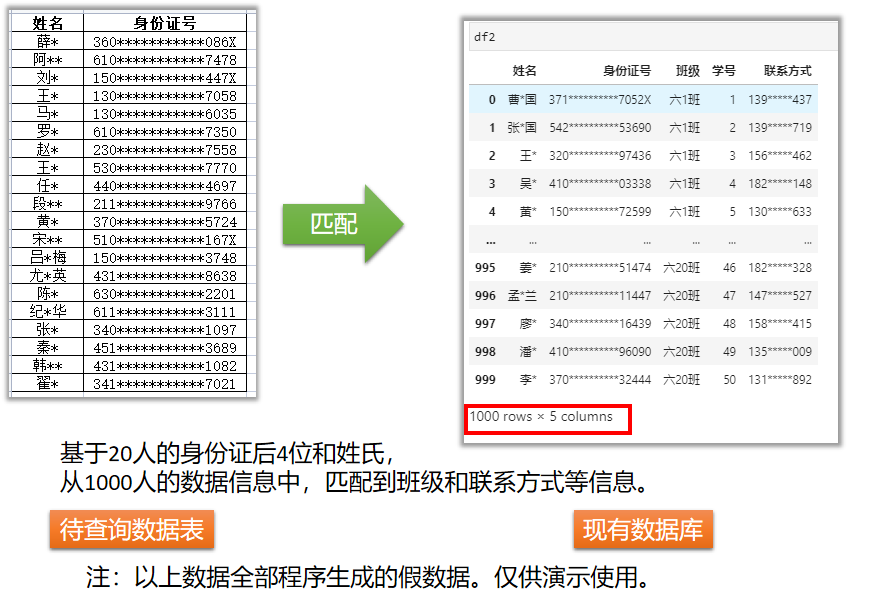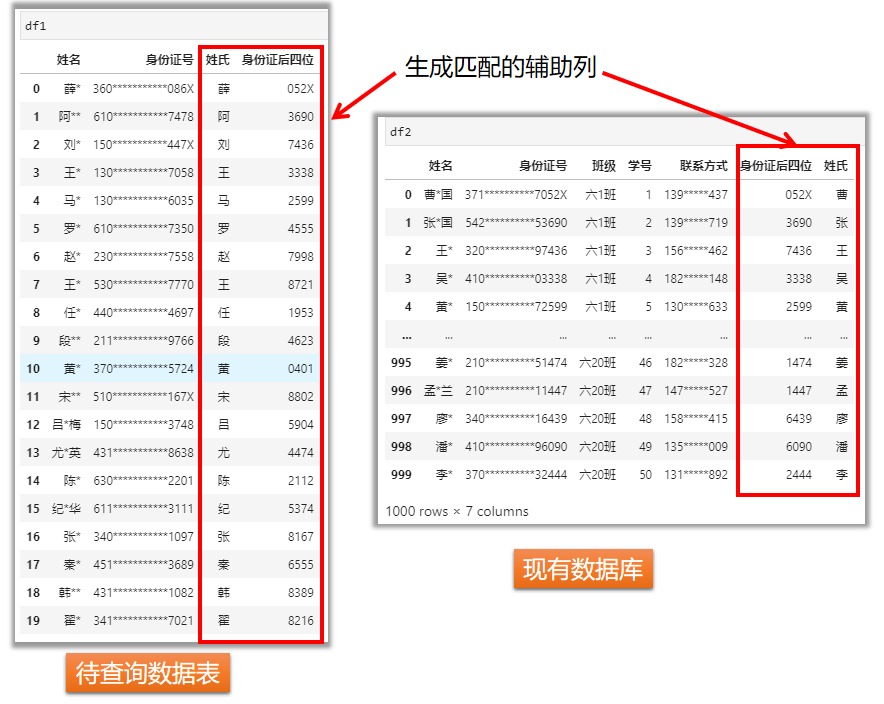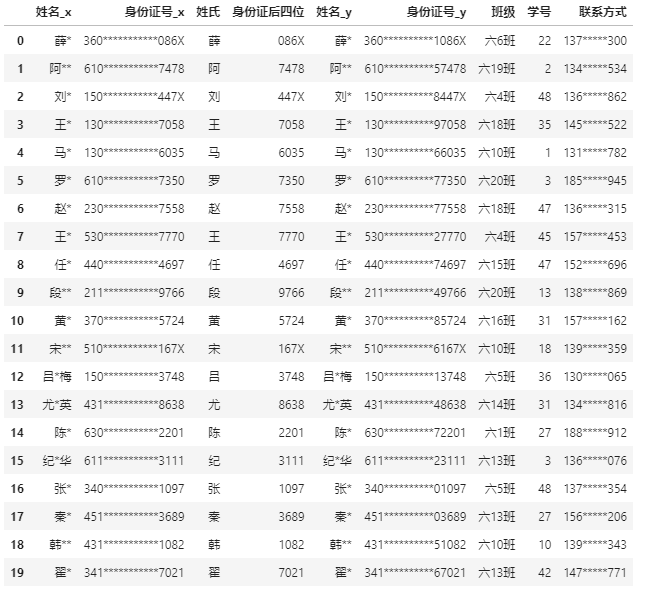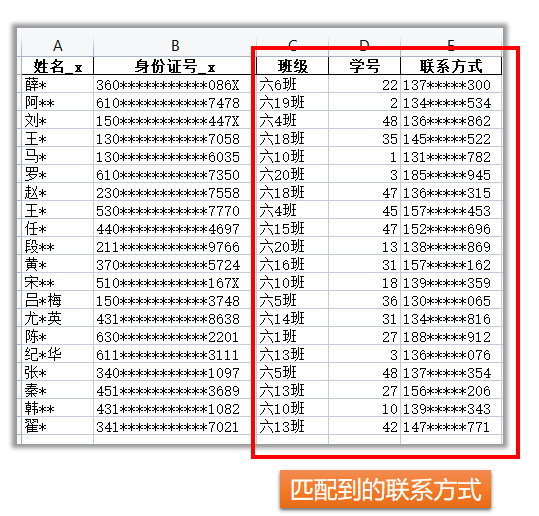Python中的`pandas`库提供了这一个问题的解决方案。代码写好后，只需关注两点：`按哪列数据匹配``要提取哪些列`，便可以解决这类问题。

## 问题描述## 1.辅助列生成

``````import pandas as pd

# 读取Excel文件

# 待查询数据表——生成辅助列：姓氏
df1['姓氏'] = df1['姓名'].str
df1['身份证后四位'] = df1['身份证号'].str[-4:]
# 数据库表——生成辅助列：编号后四位和姓氏
df2['身份证后四位'] = df2['身份证号'].str[-4:]
df2['姓氏'] = df2['姓名'].str
``````## 2.合并匹配

``````mergeDf = pd.merge(df1,df2,how='left',on=['身份证后四位','姓氏'])
``````## 3.选取另存

``````# 选取需要的列名
outColNames = [
'姓名_x',
'身份证号_x',
'班级',
'学号',
'联系方式',
]
mergeDf[outColNames].to_excel("数据匹配后的结果.xlsx",index=False)
``````